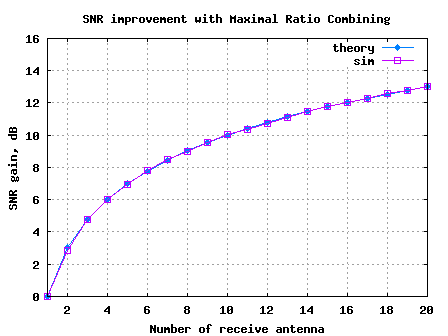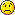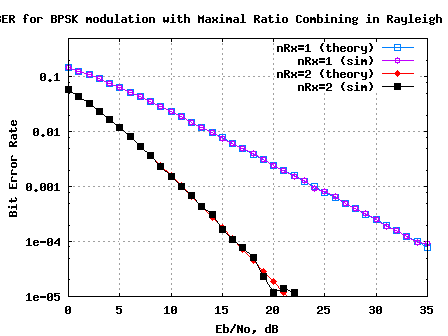- DSP log - http://www.dsplog.com -

Maximal Ratio Combining (MRC)

Posted By Krishna Sankar On September 28, 2008 @ 11:11 am In MIMO | 145 Comments

This is the third post in the series discussing receiver diversity in a wireless link. Receiver diversity is a form of space diversity, where there are multiple antennas at the receiver. The presence of receiver diversity poses an interesting problem – how do we use ‘effectively‘ the information from all the antennas to demodulate the data. In the previous posts, we discussed selection diversity  and equal gain combining (EGC) .

In this post, we will discuss Maximal Ratio Combining (MRC). For the discussion, we will assume that the channel is a flat fading Rayleigh multipath channel  and the modulation is BPSK.

## Background

We use the same constraints as defined in the Selection Diversity  and Equal Gain Combining (EGC)  post. Let me repeat the same.

1. We have N receive antennas and one transmit antenna.

2. The channel is flat fading – In simple terms, it means that the multipath channel has only one tap. So, the convolution operation reduces to a simple multiplication. For a more rigorous discussion on flat fading and frequency selective fading, may I urge you to review Chapter 15.3 Signal Time-Spreading from [DIGITAL COMMUNICATIONS: SKLAR]3. The channel experienced by each receive antenna is randomly varying in time. For the receive antenna, each transmitted symbol gets multiplied by a randomly varying complex number . As the channel under consideration is a Rayleigh channel, the real and imaginary parts of are Gaussian distributed having mean and variance .

4. The channel experience by each receive antenna is independent from the channel experienced by other receive antennas.

5. On each receive antenna, the noise has the Gaussian probability density function with

with and .

The noise on each receive antenna is independent from the noise on the other receive antennas.

6. At each receive antenna, the channel is known at the receiver.

7. In the presence of channel , the instantaneous bit energy to noise ratio at receive antenna is . For notational convenience, let us define,

.

## Maximal Ratio Combining (MRC)

where

is the channel on the receive antenna,
is the transmitted symbol and
is the noise on receive antenna.

Expressing it in matrix form, the received signal is,

, where

is the channel on all the receive antenna

is the transmitted symbol and

is the noise on all the receive antenna.

The equalized symbol is,

.

It is intuitive to note that the term,

i.e sum of the channel powers across all the receive antennas.

Note: The equations in the post refers the note on Receive diversity  by Prof. RaviRaj Adve.

## Effective Eb/No with Maximal Ratio Combining (MRC)

Earlier, we noted that in the presence of channel , the instantaneous bit energy to noise ratio at receive antenna is

.

Given that we are equalizing the channel with , with the receive antenna case, the effective bit energy to noise ratio is,

.

Effective bit energy to noise ratio in a N receive antenna case is N times the bit energy to noise ratio for single antenna case. Recall, this gain is same as the improvement which we got in Receive diversity for AWGN  caseFigure: Effective SNR with Maximal Ratio Combining in Rayleigh fading channel

## Error rate with Maximal Ratio Combining (MRC)

From the discussion on chi-square random variable , we know that, if is a Rayleigh distributed random variable, then is a chi-squared random variable with two degrees of freedom. The pdf of is
.

Since the effective bit energy to noise ratio is the sum of such random variables, the pdf of is a chi-square random variable  with degrees of freedom. The pdf of is,
.

If you recall, in the post on BER computation in AWGN , with bit energy to noise ratio of , the bit error rate for BPSK in AWGN is derived as

.

Given that the effective bit energy to noise ratio with maximal ratio combining is, the total bit error rate is the integral of the conditional BER integrated over all possible values of .

.

This equation reduces to

, where

.

Refer Equation 11.12 and Equation 11.13 in Section 11.3.1 Performance with Maximal Ratio Combining in [DIG-COMM-BARRY-LEE-MESSERSCHMITT].  Again, I do not know the proof## BER Simulation Model

The Matlab/Octave script performs the following

(a) Generate random binary sequence of +1′s and -1′s.

(b) Multiply the symbols with the channel and then add white Gaussian noise.

(c) Chose that receive path, equalize the received symbols per maximal ratio combining

(d) Perform hard decision decoding and count the bit errors

(e) Repeat for multiple values of and plot the simulation and theoretical results.Figure: BER plot for BPSK in Rayleigh channel with Maximal Ratio Combining

## Reference

[DIG-COMM-BARRY-LEE-MESSERSCHMITT] Digital Communication: Third Edition, by John R. Barry, Edward A. Lee, David G. MesserschmittURL to article: http://www.dsplog.com/2008/09/28/maximal-ratio-combining/

URLs in this post:

 equal gain combining (EGC): http://www.dsplog.com/2008/09/19/equal-gain-combining/

 Rayleigh multipath channel: http://www.dsplog.com/2008/07/14/rayleigh-multipath-channel/

 Matlab/Octave script for plotting effective SNR with Maximal Ratio Combining in Rayleigh channel: http://www.dsplog.com/db-install/wp-content/uploads/2008/09/script_maximal_ratio_combining_effective_snr.m

 chi-square random variable: http://www.dsplog.com/2008/07/28/chi-square-random-variable/#twodegree

 chi-square random variable: http://www.dsplog.com/2008/07/28/chi-square-random-variable/#mdegree

 BER computation in AWGN: http://www.dsplog.com/2007/08/05/bit-error-probability-for-bpsk-modulation/